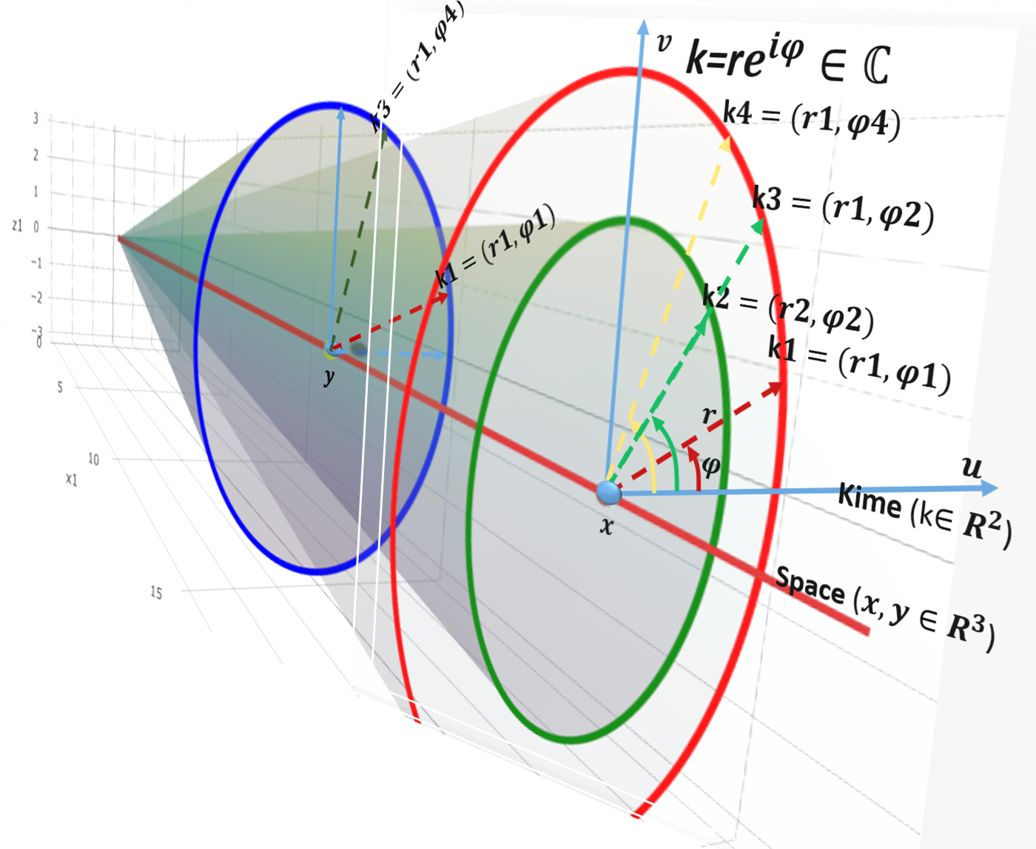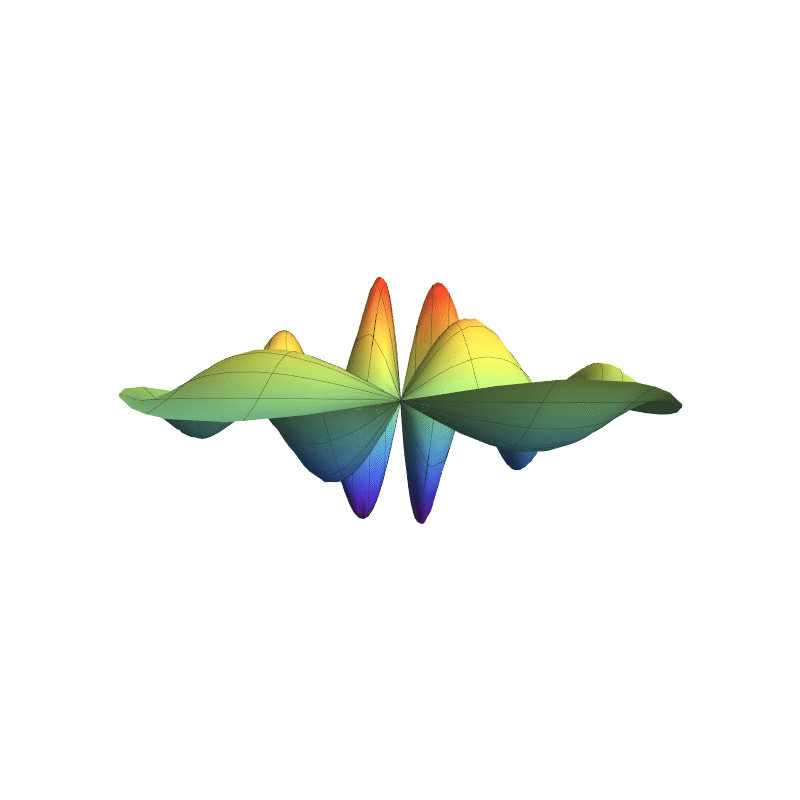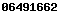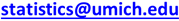##### Spacekime: The 5D universe of 3D-space and 2D-complex-time (kime)
###### Perceptions of the natural world are dramatically changed by examining commonly observed phenomena in higher dimensions Have you ever asked any of the following questions?

• What is time and how are time-measurements different from space-locations?
• What is an event and how is it characterized?
• Why can't we simultaneously measure the position and the momentum of a moving particle with perfect accuracy?
• Is it possible that in reality the universe is higher-dimensional, yet we can only perceive a lower-dimensional projection (a silhouette) of an actual holographic universe?
• What is a probability distribution? Can we observe the entire probability distribution or only see samples of finite sizes?
• What is the Kaluza-Klein Theory and why is it important?
• What would the universe look like if the 4D space-time space we experience is simply a projection of a higher dimensional space?
• What is Heisenberg's uncertainty principle and how is it related to distribution theory, stochastic, and deterministic dynamics?
• The classical first and second fundamental laws of probability theory (CLT and LLN) provide asymptotic results about observed sample statistics and their convergence to population process characteristics. Under what conditions, small-samples may still lead to reliable scientific inference?
• Do ultrahyperbolic partial differential equations (like the wave equation) have solutions in multiple time dimensions, and if so, what are the dynamics of potential functions solving such PDEs (e.g., wavefunctions)?### Overview

The SOCR group at the University of Michigan is developing a novel theoretical foundation to extend the notion of time to the complex plane. This approach lifts the concept of time from a positive real number representing event ordering to a 2D complex-time (kime) comprising a pair of coordinates - time (t) and phase (φ). This approach enables powerful data-driven analytical strategies for large longitudinal data. The 5D spacekime analytics utilize information measures, entropy, KL divergence, PDEs, Dirac’s bra-ket operators, and the Fourier transform. This data science fundamentals research project explores time-complexity and inferential uncertainty in modeling, analyzing, and interpreting large, heterogeneous, multi-source, multi-scale, incomplete, incongruent, and longitudinal data.In 4D spacetime, classical time-series are represented as real-valued functions defined over the positive real time domain. In the 5D spacekime manifold, time-series (curves) extend to kime-series, which are represented geometrically as surfaces. This animation illustrates the surface corresponding to one such kime-series at a fixed spatial location. At any given kime, i.e., for a pair of arguments kime-magnitude (t) and the kime-phase (φ), the height of the kime-surface represents the intensity of the kime-series and is coded in rainbow color. Notice the 2D parametric kime grid superimposed on the surface of the kime-series. There are kime-phase aggregating operators that can be used to transform standard time-series curves to spacekime kime-surfaces. These kimesurfaces that can be modeled, interpreted, analyzed, and predicted using advanced spacekime analytics.

### Kime-series (kimesurfaces)

In many disciplines like music, economics, biomedicine, and health sciences, the flow of data and information is time-dependent. This includes the longitudinal progression of events, frequencies, motion, states, etc. In the 5D spacekime manifold, these time-courses, temporal events, or time-series, are actually complex-time surfaces (kimesurfaces) whose realizations in the lower-dimensional 4D spacetime are observed simply as curves over time.
The gif animations above illustrate the relation between the commonly observed longitudinal time-series and their reconstructed kimesurface counterparts. Note that observable time-series are just intersections of the more general kimesurfaces with 2D leaf foliation manifolds, e.g., planes. Specifically, the third animation shows an idealized (over-simplified and smooth) version of a complex-valued and kime-indexed fMRI at one spatial location. Note that the domain is indexed by the kime magnitude (time) and phase (direction), and the amplitude and the phase of the fMRI intensity are encoded respectively by the kimesurface height (z-axis) and its color (RGB).

For a more concrete example, imagine a symphony performance in a concert hall. At roughly the same time and the same spatial location (ignore periphery seats and bleacher rows for now), all attendees (observers) receive the same longitudinal input (e.g., temporal audio-visual-tactile stimuli). However, at the end of the performance, all attendees leave the concert hall with relatively different experiences, distinct impressions, and unique opinions about the adventure. At each instance of time, all observers share the same spacetime location, but their differential experiences correspond to random sampling through the kime-phase space (Φ), which explains their uniquely individual encounters. After the performance concludes, communication and stratification of the individual experiences lead to review(s) reflecting the “overall consensus” capturing the joint experience, performance quality, impact, and value of the encounter. In other words, kime-averaging of all impressions gives us a yardstick measure of overall performance quality that can be used to compare, contrast, or calibrate other similar past or future performances.

##### Complex Time (kime)

Over 100 years ago, the ideas of many polymaths materialized into a unifying notion of an integrated 4D Minkowski space-time. Kaluza and Klein were the first to ask what may be the implications of adding a special tiny (non-traversable) fifth dimension. Later the work of Wesson, Bars, and the 5D space-time-matter consortium generalized this idea. The SOCR team is now extending the interpretation of the universe as a 5D space-kime manifold where time is no longer simply a positive unidirectional concept, but a complete field isomorphic to the complex numbers. This leads to interesting extensions of classical concepts like time, events, and spacetime metric, to their more general counterparts, kime, kevents, and spacekime metric tensor. This generalization resolves some of the problems of time and enables the formulation of time-dependent properties as process characteristics derived using the 2D kime. Additionally, the quantum mechanical notions of particle and wavefunction are extended to datasets and data science analytics.

##### Spacekime details

The time-complexity and inferential-uncertainty textbook provides detailed formulation of the spacekime manifold and its applications to data science and longitudinal health analytics.

##### Spacekime data analytics

The lifting of the 4D spacetime into the 5D spacekime manifold has profound implications on the collection, modeling, interpretation, and analytics of all longitudinal information.

Two specific implications include:

• Reduction of the need for large-samples to estimate specific population characteristics; and
• Improvement of the subsequent data analytics performed on spacekime-transformed data.
Learn more about the spacekime project at www.spacekime.org or by contacting SOCR’s Director, Prof. Ivo Dinov.
##### Spacekime Project Goals

This project aims to answer some deep and fundamental questions like:

• How to translate centuries of fundamental mathematical and physics ideas into data science?
• Can large-sample theory be replaced by small-samples with some prior knowledge?
• Is the universe really four-dimensional?
• What if there is no arrow of time and we can traverse spacetime in any direction?
• Would lifting the spacetime dimension lead to differences in the interpretation of information and improvement of the resulting data-driven inference, prediction, or clustering?
##### Spacekime Publications

See the SOCR Publications Website

SOCR Resource Visitor number